Test: Transportation Engineering- 2

# Test: Transportation Engineering- 2

Test Description

## 15 Questions MCQ Test GATE Civil Engineering (CE) 2023 Mock Test Series | Test: Transportation Engineering- 2

Test: Transportation Engineering- 2 for Civil Engineering (CE) 2023 is part of GATE Civil Engineering (CE) 2023 Mock Test Series preparation. The Test: Transportation Engineering- 2 questions and answers have been prepared according to the Civil Engineering (CE) exam syllabus.The Test: Transportation Engineering- 2 MCQs are made for Civil Engineering (CE) 2023 Exam. Find important definitions, questions, notes, meanings, examples, exercises, MCQs and online tests for Test: Transportation Engineering- 2 below.
Solutions of Test: Transportation Engineering- 2 questions in English are available as part of our GATE Civil Engineering (CE) 2023 Mock Test Series for Civil Engineering (CE) & Test: Transportation Engineering- 2 solutions in Hindi for GATE Civil Engineering (CE) 2023 Mock Test Series course. Download more important topics, notes, lectures and mock test series for Civil Engineering (CE) Exam by signing up for free. Attempt Test: Transportation Engineering- 2 | 15 questions in 45 minutes | Mock test for Civil Engineering (CE) preparation | Free important questions MCQ to study GATE Civil Engineering (CE) 2023 Mock Test Series for Civil Engineering (CE) Exam | Download free PDF with solutions
 1 Crore+ students have signed up on EduRev. Have you?
Test: Transportation Engineering- 2 - Question 1

### The radius of a horizontal circular curve on a highway is 120 m. The design speed is 60 km/hour, and the design coefficient of lateral friction between the tyre and the road surface is 0.15. The estimated value of superelevation required (if full lateral friction is assumed to develop), and the value of the coefficient of friction needed (if no superelevation is provided) will, respectively, be

Detailed Solution for Test: Transportation Engineering- 2 - Question 1

Given ⇒ R = 120 m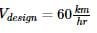f = 0.15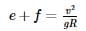If full lateral friction is assumed to develop, then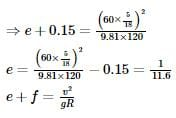If, e = 0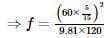= 0.24

Test: Transportation Engineering- 2 - Question 2

### The critical flow ratios for a three-phase signal are found to be 0.30, 0.25, and 0.25. The total time lost in the cycle is 10 s. Pedestrian crossings at this junction are not significant. The respective Green times (expressed in seconds and rounded off to the nearest integer) for the three phases are

Detailed Solution for Test: Transportation Engineering- 2 - Question 2

Y = y1 + y2 + y3

= 0.30 + 0.25 + 0.25= 0.80

Now Total lost time

L = 10 sec (given)

∴ Optimum cycle time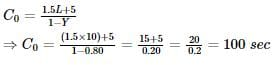Now green times are calculated by,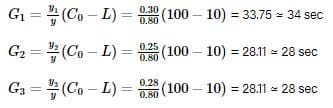*Answer can only contain numeric values
Test: Transportation Engineering- 2 - Question 3

### A motorist traveling at 100 km/h on a highway needs to take the next exit, which has a speed limit of 50 km/h. The section of the roadway before the ramp entry has a downgrade of 3% and the coefficient of friction ( f ) is 0.35. In order to enter the ramp at the maximum allowable speed limit, the braking distance (expressed in m) from the exit ramp is _________.

Detailed Solution for Test: Transportation Engineering- 2 - Question 3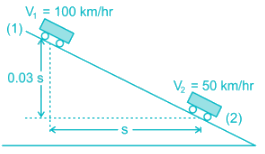Total energy lost between point (1) and (2)

= Work done by frictional force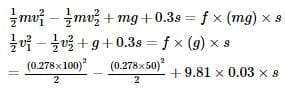= 0.35 × 9.81 × s

289.815 = 3.1392 s

s = 92.32 m

*Answer can only contain numeric values
Test: Transportation Engineering- 2 - Question 4

The relation between speed (in km/h) and density (number of vehicles / km) for a traffic stream on a road is = 70 – 0.7k. The capacity on this road is _______________ vph (vehicles/hour).

Detailed Solution for Test: Transportation Engineering- 2 - Question 4

Traffic volume,

q = uk

q = (70 – 0.7 k) k= 70k – 0.7 k2

Maximum possible volume is capacity of road.

For maximum volume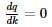⇒ 70 – 1.4 k = 0

⇒ k = 50 Veh /km

Hence, capacity

C = (70 – 0.7 × 50) × 50

C = 1750 Veh/hr

Test: Transportation Engineering- 2 - Question 5

During a forensic investigation of pavement failure, an engineer reconstructed the graphs P, Q, R and S, using partial and damaged old reports.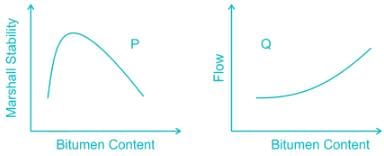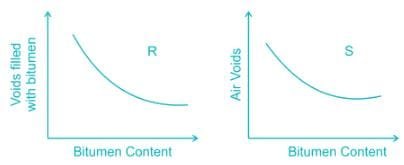Theoretically plausible correct graphs according to the 'Marshall mixture design output' are

Detailed Solution for Test: Transportation Engineering- 2 - Question 5

The graph between VFB and Bitumen content is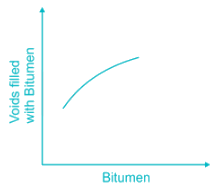Test: Transportation Engineering- 2 - Question 6

A superspeedway in New Delhi has among the highest super-elevation rates of any track on the Indian Grand Prix circuit. The track requires drivers to negotiate turns with a radius of 335 m and 33° banking. Given this information, the coefficient of side friction required in order to allow a vehicle to travel at 320 km/h along the curve is:

Detailed Solution for Test: Transportation Engineering- 2 - Question 6

R = 335 m

V = 320 km/hr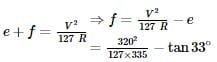= 1.761

*Answer can only contain numeric values
Test: Transportation Engineering- 2 - Question 7

The penetration value of a bitumen sample tested at 25°C is 80. When this sample is heated to 60°C and tested again, the needle of the penetration test apparatus penetrates the bitumen sample by d mm. The value of CANNOT be less than _______________ mm.

Detailed Solution for Test: Transportation Engineering- 2 - Question 7

The penetration value is 1/10th of the bitumen sample grade.

The penetration value of 80 means the needle of the penetration test apparatus penetrates the bitumen sample by 80/10= 8 mm

Test: Transportation Engineering- 2 - Question 8

In the context of the IRC 58-2011 guidelines for rigid pavement design, consider the following pair

of statements.

I: Radius of relative stiffness is directly related to the modulus of elasticity of concrete and inversely

related to Poisson's ratio.

II: Radius of relative stiffness is directly related to the thickness of slab and modulus of subgrade

reaction.

Which one of the following combinations is correct?

Detailed Solution for Test: Transportation Engineering- 2 - Question 8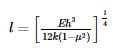As μ increases then 1 – μ2 decreases.

∴ Relative stiffness also increases

∴ Statements 1 is false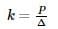Hence, Statement 2 is false.

*Answer can only contain numeric values
Test: Transportation Engineering- 2 - Question 9

While traveling along and against the traffic stream, a moving observer measured the relative flows as 50 vehicles/hr and 200 vehicles/hr, respectively. The average speeds of the moving observer while traveling along and against the stream are 20 km/hr and 30 km/hr, respectively. The density of the traffic stream (expressed in vehicles/km) is

Detailed Solution for Test: Transportation Engineering- 2 - Question 9

Solution: q = traffic flow

k = traffic density

Vw = velocity of test vehicle along the stream

Va  = velocity of test vehicle against the stream

⇒ q – kVw = 50

q – k × 20 = 50

q + kVa = 200

q + k × 30 = 200

Solving equation (i) and (ii), we get

k = 3 Veh/km

*Answer can only contain numeric values
Test: Transportation Engineering- 2 - Question 10

The radii of the relative stiffness of the rigid pavement P and Q are denoted by IP and IQ respectively. The geometric and material properties of the concrete slab and underlying soil are given below.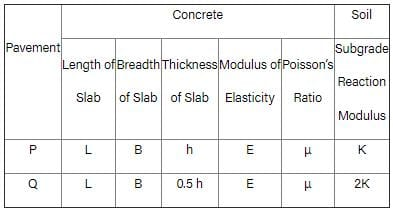The ratio (up to one decimal place) of IP / IQ is _____.

Detailed Solution for Test: Transportation Engineering- 2 - Question 10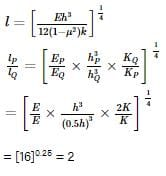Test: Transportation Engineering- 2 - Question 11

In Marshall method of mix design, the coarse aggregate, fine aggregate, fines and bitumen having respective values of specific gravity 2.60, 2.70, 2.65 and 1.01, are mixed in the relative proportions (% by weight) of 55.0, 35.8, 3.7 and 5.5 respectively. The theoretical specific gravity of the mix and the effective specific gravity of the aggregates in the mix respectively are:

Detailed Solution for Test: Transportation Engineering- 2 - Question 11

Theoretical specific gravity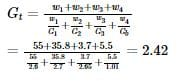Effective specific gravity of aggregates (coarse + fine) is given by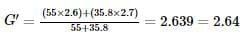Test: Transportation Engineering- 2 - Question 12

Two cars P and Q are moving in a racing track continuously for two hours. Assume that no other vehicles are using the track during this time. The expressions relating the distance traveled d (in km) and time t (an hour) for both the vehicles are given as

P (d) = 60t

Q (d) = 60t2

Within the first one hour, the maximum space headway would be

Detailed Solution for Test: Transportation Engineering- 2 - Question 12

P: d = 60t

Q: d = 60t2

Space Headway (S) = 60t2 – 60t

For space headway to be max,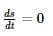120t – 60 = 0

⇒ 60(2t – 1) = 0

⇒ t = ½ hr

= 60 × ½ - 60 × (½)2

= 60 × ½ - 60 × ¼

= 30 – 15 = 15 km

*Answer can only contain numeric values
Test: Transportation Engineering- 2 - Question 13

A sign is required to be put up asking drivers to slow down to 30 km/h before entering Zone Y (see figure). On this road, vehicles require 174 m to slow down to 30 km/h (the distance of 174 m includes the distance traveled during the perception-reaction time of drivers). The sign can be read by 6/6 vision drivers from a distance of 48 m. The sign is placed at a distance of x m from the start of Zone Y so that even a 6/9 vision driver can slow down to 30 km/h before entering the zone. The minimum value of x is _______________ m.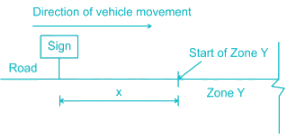Detailed Solution for Test: Transportation Engineering- 2 - Question 13

For a driver having 6/6 vision can see the board from distance 48 m.

For a driver having 6/9 vision can see the board from  a of distance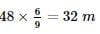Vehicle requires 174 m to slow down to 30 km/hr.

So minimum distance of X from the start of Y zone

= 174 – 32

= 142 m

Hence, the minimum value of X = 142 m

*Answer can only contain numeric values
Test: Transportation Engineering- 2 - Question 14

The acceleration-time relationship for a vehicle subjected to non-uniform acceleration is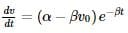where is the speed in m/s, is the time in s, α and β are parameters, and v0 is the initial speed in m/s. If the accelerating behavior of a vehicle, whose driver intends to overtake a slow-moving vehicle ahead, is described as,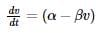Considering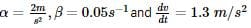at t = 3 s, the distance (in m) traveled by the vehicle in 35 s is ___.

Detailed Solution for Test: Transportation Engineering- 2 - Question 14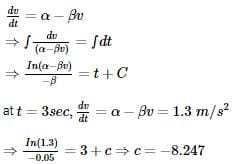∴ In (2 – 0.05 × v) = (t – 8.247) – (– 0.05)

⇒ 2 – 0.05 × v = 1.51 e-0.05t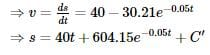∴ at t = 0, s = 0 ⇒ c’ = – 604.15

Hence, s = 40t + 604.15 e-0.05t – 604.15

at t = 35 sec

s = 40 × 35 + 604.15 e-0.05 × 35 – 604.15

s = 900.84 m

Test: Transportation Engineering- 2 - Question 15

The queue length (in a number of vehicles) versus time (in seconds) plot for an approach to a signalized intersection with the cycle length of 96 seconds is shown in the figure (not drawn to scale).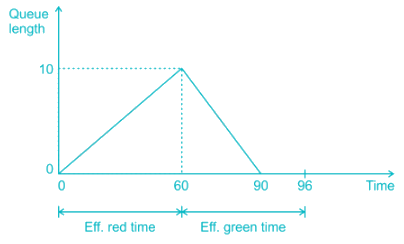At time = t = 0, the light has just turned red. The effective green time is 36 seconds, during which vehicles discharge at the saturation flow rate, s (in vph). Vehicles arrive at a uniform rate, v (in vph), throughout the cycle. Which one of the following statements is TRUE?

Detailed Solution for Test: Transportation Engineering- 2 - Question 15

Vehicle arrived upto 60 sec (Red time) = 10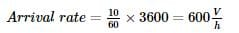V = 600 V/h

Between = 60 second to 90 second total vehicle departed

= Vehicle arrived upto 60 second + Vehicle arriving between 60 sec to 90 sec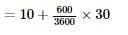= 10 + 5

= 15

So, departure rate = Saturation flow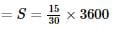S = 1800 v/h

Average delay time is given by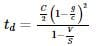C = 96 seconds

g = 96 – 60 = 36 seconds

V = 600 Vph

S = 1800 Vph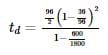td = 28.125 seconds

## GATE Civil Engineering (CE) 2023 Mock Test Series

27 docs|296 tests
Information about Test: Transportation Engineering- 2 Page
In this test you can find the Exam questions for Test: Transportation Engineering- 2 solved & explained in the simplest way possible. Besides giving Questions and answers for Test: Transportation Engineering- 2, EduRev gives you an ample number of Online tests for practice

## GATE Civil Engineering (CE) 2023 Mock Test Series

27 docs|296 tests(Scan QR code)